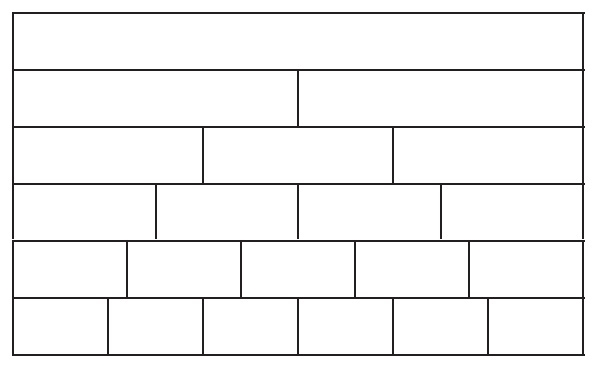By: Chet Delani & Mary Saltus

Helping students build an understanding of fraction concepts is a challenge in the intermediate and middle grades. The pictorial representation is a critical tool for making the connection between the concept and the procedure. Conceptual understanding occurs when students can explain why the procedure works, showing that they have assimilated or integrated this understanding into their basic knowledge of fractions.

The premise of Fraction Games & Activities with Dice! is to provide students with activities that connect the concept with the procedure. The ten scripted mini-lessons provide opportunities for students to engage in explaining this connection, while the dice activities allow them to practice applying the concepts.

## Understanding 1 as the Identity Element

The basis for understanding equivalent fractions and renaming fractions is the concept of 1 as the identity element. If students understand that any number times one equals the original number (for example, 5 x 1 = 5 or a x 1 = a) then the next sequential concept would be to explore the idea of a/a that can be presented as 44 = 55 = 33 .

Once this concept is realized students can apply it to equivalent fractions. The concept suggests that if 12 x 1 = 12, then 12 x 55 = 12 , which can also be named as 510 using the multiplication algorithm.

Presented with the visual model below, students can see that 13 is same amount as 26 and that 22 is the same amount as 66 or 44 and so on. One is the same no matter how many parts it is partitioned into.Common instruction often follows the pictorial representation to renaming fractions with a procedure. The two are not always connected and are often viewed by the student as two disparate experiences. The procedure relies on memory rather than providing an understanding of why (a × n)(b × n)=anbn.

The procedure renames a fraction by multiplying both the numerator and the denominator by one. To produce different names for the fraction you multiply both the numerator and the denominator by the same number.

## What does this mean? Why does this work?

The sample activities from Fraction Games & Activities with Dice! included with this article help students understand how and why multiplying 13 by 33 results in equivalency. The mini-lesson introduces 1 as the identity element in renaming fractions and provides a dice activity that reinforces this understanding. The lesson provides students an opportunity to explain their understanding, which is crucial in developing a conceptual understanding.

Following this mini-lesson with the Finding Ones Dice Activity provides students an opportunity to reinforce their understanding that any fraction where the denominator and numerator are the same is a fraction that is always equal to 1.

## Conclusion

Helping students make the connection between the concept of equivalent fractions and the associated procedure ensures the deep conceptual understanding that they need. The lessons and activities in Fraction Games & Activities with Dice! provide experiences with fraction concepts that help students build their knowledge of this and other critical fraction concepts.